# RBSE Maths Class 9 Chapter 7: Important Questions and Solutions

RBSE Maths Chapter 7 – Congruence and Inequalities of Triangles Class 9 Important questions and solutions are provided here. The important questions and solutions of Chapter 7 are available at BYJU’S and have detailed explanations. Also, RBSE Class 9 solutions are useful for the students to understand each and every concept, without any complexity.

Chapter 7 of the RBSE Class 9 Maths contains major concepts of triangles, namely congruence of triangles, inequalities with respect to triangles and related theorems. All these concepts are useful for the students for their higher classes too. Hence, after practicing all these important questions, students will be able to prove certain statements in geometry in relation with the congruence rules.

### RBSE Maths Chapter 7: Exercise 7.1 Textbook Important Questions and Solutions

Question 1: In ΔABC and ΔPQR, ∠A = ∠Q and ∠B = ∠R. Which side of ΔPQR should be equal to the side AB of ΔABC, so that two triangles become congruent? Give reason to your answer.

Solution:

In ΔABC and ΔPQR,

∠A = ∠Q and ∠B = R

By ASA congruence criterion,

AB should be equal to QR so that the given two triangles will be congruent.

AB = QR

Thus, ΔABC ≅ ΔPQR

Question 2: In triangles ABC and PQR, ∠A = ∠Q and ∠B = R. Which side of ΔPQR should be equal to side BC of ΔABC, so that two triangles are congruent? Give reason to your answer.

Solution:

In ΔABC and ΔPQR,

∠A = ∠Q and ∠B = R

If two angles of two triangles are congruent, then the corresponding third angle equals both the triangles.

Therefore, ∠C = ∠P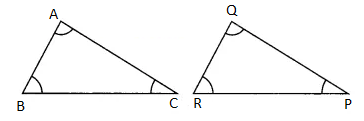That means, PR is equal to BC.

Hence, ΔABC ≅ ΔPQR by ASA criterion.

Question 3: If two sides and one angle of a triangle is equal to the two sides and one angle of another triangle, then two triangles should be congruent. Is this statement true? Why?

Solution:

No, by SAS congruence criterion, two triangles are said to be congruent if two sides one included angle of a triangle is equal to the corresponding two sides and one included angle.

Question 4: If two angles and one side of a triangle is equal to the two angles and one side of another triangle, then triangles should be congruent. Is this statement true? Why?

Solution:

No, by ASA congruence criterion, two triangles are said to be congruent if two angles one included side of a triangle is equal to the corresponding two angles and one included side.

Question 5: It is given that ΔABC ≅ ΔRPQ. Is BC = QR true? Why?

Solution:

Given,

ΔABC ≅ ΔRPQ

We know that corresponding sides of congruent triangles are equal.

Thus,

AB = RP

BC = PQ

CA = QR

Therefore, BC = QR is not true.

Question 6: If ΔPQR ≅ ΔEDF, then is this true PR = EF? Give reason to your answer.

Solution:

Given,

ΔPQR ≅ ΔEDF

We know that corresponding sides of congruent triangles are equal.

Thus,

PQ = ED

QR = DF

RP = FE

Therefore, PR = EF.

Question 7: In the figure, diagonal AC of quadrilateral ABCD is the bisector of ∠A and ∠C. Prove that: AB = AD and CB = CD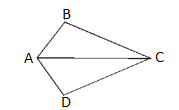Solution:

∠ACB = ∠ACD (given)

∠BAC = ∠DAC (given)

AC = AC (common side)

By ASA congruence criterion,

By CPCT,

CB = CD

Hence proved.

Question 8: In the figure, in quadrilateral ADBC, ∠ABC = ∠ABD and BC = BD, then prove that: ΔABC ≅ ΔABD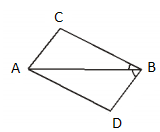Solution:

In ΔABC and ΔABD,

∠ABC = ∠ABD (given)

BC = BD (given)

AB = AB (common)

By SAS congruence criterion,

ΔABC ≅ ΔABD

Hence proved.

### RBSE Maths Chapter 7: Exercise 7.2 Textbook Important Questions and Solutions

Question 9: In the figure AB = AC and ∠B = 58° then find the value of ∠A.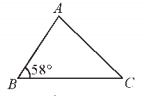Solution:

Given,

∠B = 58°

AB = AC

Angles corresponding to the equal sides are also equal.

∠B = ∠C = 58°

∠A + ∠B + ∠C = 180°

∠A + 58° + 58° = 180°

∠A = 180° – 116°

∠A = 64°

Question 10: In the figure, AD = BD and ∠C = ∠E, then prove that BC = AE.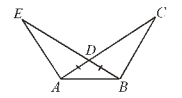Solution:

Given that,

∠C = ∠E

∠ADE = ∠BDC (vertically opposite angles)

By AAS congruence criterion,

ΔAED ≅ ΔBCD

By CPCT,

AE = BC

Hence proved.

Question 11: If AD is the median of an isosceles triangle ABC and ∠A = 120° and AB = AC, then find the value of ∠ADB.

Solution:

According to the given,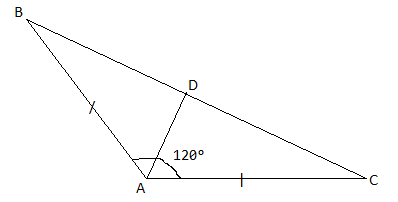In ΔABC,

AB = AC (given)

Let us assume ∠B = ∠C = x

By the angle sum property,

∠A + ∠B + ∠C = 180°

120° + x + x = 180°

2x = 180° – 120°

x = 60°/2 = 30°

∠ADB = 180° – (∠B + 1/2 ∠A)

= 180° – (60° + 30°)

= 180° – 90°

= 90°

Question 12: If the bisector of any angle of a triangle also bisects the opposite side, then prove that the triangle is an isosceles triangle.

Solution:

Let ABC be the isosceles triangle such that AB = AC.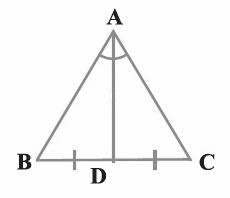BD = CD (Given)

∠ABD = ∠ACD (AD is a common side, angles opposite equal sides are equal)

By AAS congruence criterion,

△ABD ≅ △ACD

By CPCT,

AB = AC

Therefore, ABC is an isosceles triangle.

Question 13: In the figure, AB = AC and BE = CD, then prove that AD = AE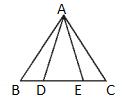Solution:

Given that,

AB = AC

BE = CD

∠B = ∠C

By SAS congruence criterion,

ΔACD ≅ ABE

By CPCT,

Hence proved.

Question 14: E and F are two points on sides AD and BC, respectively of square ABCD such that AF = BE. Show that:

(i) ∠BAF = ∠ABE

(ii) BF = AE

Solution:

According to the given, square ABCD is: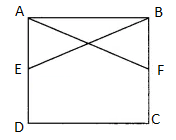In ΔAEB and ΔAFB,

AF = BE

∠BAE = ∠ABF = 90° (ABCD is a square)

AB = AB (common side)

By SAS congruence criterion,

ΔEAB ≅ ΔFBA

By CPCT,

∠FAB = ∠EBA

BF = AE

Question 15: The bisectors of angles B and C of an isosceles triangle with AB = AC, intersect each other at point O. Produce BO upto point M. Prove that ∠MOC = ∠ABC.

Solution: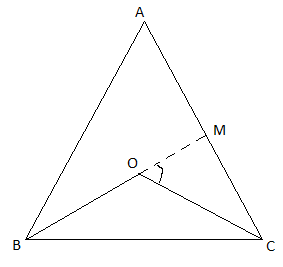In triangle ABC,

AB = AC (given)

∠B = ∠C (given)

Consider triangle BOC,

∠MOC = ∠OBC + ∠OCB

= (1/2) [2∠OBC + 2∠OCB]

= (1/2)(∠B + ∠C)

= (1/2) (∠B + ∠B)

= (1/2) × 2∠B

= ∠B

∠MOC= ∠ABC

Hence proved.

### RBSE Maths Chapter 7: Exercise 7.3 Textbook Important Questions and Solutions

Students can refer to the detailed solutions of this exercise here. These solutions contain required statements to be given as reasons so that students can understand easily.

### RBSE Maths Chapter 7: Additional Important Questions and Solutions

Question 1: Which one of the following is not the condition of congruence of triangles.

(a) SAS

(b) ASA

(c) SSA

(d) SSS

Solution:

The congruence rules for two triangles are SSS, SAS, ASA, AAS and RHS. There is no such rule of SSA for congruence of triangles.

Question 2: In ΔABC, BC = AB and ∠B = 80°, then ∠A is equal to:

(a) 80°

(b) 40°

(c) 50°

(d) 100°

Solution:

Given that,

In triangle ABC, BC = AB

Thus,

∠A = ∠C

By the angle sum property,

∠A + ∠B + ∠C = 180°

∠A + 80° + ∠A = 180°

2∠A = 180° – 80° = 100°

∠A = 100°/2 = 50°

Question 3: In ΔPQR, ∠R = ∠P and QR = 4 cm and PR = 5 cm, then length of PQ is:

(a) 4 cm

(b) 5 cm

(c) 2 cm

(d) 2.5 cm

Solution:

Given that,

In ΔPQR,

QR = 4 cm and PR = 5 cm

∠R = ∠P

Triangle PQR is an isosceles triangle.

Therefore,

QR = PQ = 4 cm

Question 4: Lengths of two sides of a triangle are 5 cm and 1.5 cm. Following cannot be the length of its third side

(a) 3.6 cm

(b) 4.1 cm

(c) 3.8 cm

(d) 3.4 cm

Solution:

We know that the sum of two sides of a triangle should be greater than or equal to the third side.

Thus,

1.5 + 3.6 = 5.1 cm > 5 cm

1.5 + 4.1 = 5.6 cm > 5 cm

1.5 + 3.8 = 5.3 cm > 5 cm

1.5 + 3.4 = 4.9 < 5 cm

Therefore, 3.4 cm cannot be the length of the third side.

Question 5: If AB = QR, BC = PR and CA = PQ, then:

(a) ΔABC ≅ ΔPQR

(b) ΔCBA ≅ ΔPRQ

(c) ΔBAC ≅ ΔRPQ

(d) ΔPQR ≅ ΔBCA

Solution:

Given that,

AB = QR

BC = PR

CA = PQ

To represent the congruence of two triangles take the vertices with respect to the ratio of sides.

By CPCT,

ΔCBA ≅ ΔPRQ

Question 6: It is given that ΔABC ≅ FDE and AB = 5 cm, ∠B = 40° and ∠A = 80°. Which one of the following is true?

(a) DF = 5 cm, ∠F = 60°

(b) DF = 5 cm, ∠E = 60°

(c) DE = 5 cm, ∠E = 60°

(d) DE = 5 cm, ∠D = 40°

Solution:

Given,

ΔABC ≅ FDE

By CPCT,

AB = FD = 5 cm

∠B = ∠D = 40°

∠A = ∠F = 80°

∠C = ∠E

In triangle ABC,

∠A + ∠B + ∠C = 180°

80° + 40° + ∠C = 180°

∠C = 180° – 120° = 60°

Therefore, DF = 5 cm and ∠E = 60°

Question 7: In triangle ABC and PQR, AB = AC, ∠C = ∠P and ∠B = ∠Q. These triangles are:

(a) Isosceles but not congruent

(b) Isosceles and congruent

(c) Congruent but not isosceles

(d) Neither congruent nor isosceles

Solution:

Given,

AB = AC ….(i)

∠C = ∠P and ∠B = ∠Q

Therefore, PR = QR ….(ii)

From (i) and (ii),

Two triangles are isosceles.

To be congruent, there should be at least one included side that is equal in both the triangles.

Hence, they are isosceles but not congruent.

Question 8: In right triangle ABC, if ∠C is a right angle then longest side will be:

(a) AB

(b) 5C

(c) CA

(d) None of these

Solution:

Given that, in the right triangle ABC, ∠C is the right angle.

Side opposite to ∠C is AB, which is hypotenuse.

We know that hypotenuse is the longest side in the right triangle.

Therefore, AB is the longest side.

Question 9: The difference of any two sides of a triangle to its third side is:

(a) more

(b) same

(c) less

(d) half

Solution:

We know that the sum of any two sides of a triangle is greater than or equal to the third side.

From this, it is clear that the difference between any two sides of a triangle is less than its third side.

Question 10: In triangles ABC and DEF, AB = FD and ∠A = ∠D. Two triangles are congruent by SAS rule if:

(a) BC = EF

(b) AC = DE

(c) AC = EF

(d) BC = FE

Solution:

Given that,

AB = FD

∠A = ∠D

By SAS congruence rule,

Two sides and one included angle should be equal.

Therefore, AC = DE

Question 11: If in ΔABC, AB = AC and ∠A < 60°, then write the relation between BC and AC.

Solution:

Given that in triangle ABC,

AB = AC and ∠A < 60°

That means, ABC is an isosceles triangle.

∠B = ∠C

Therefore, BC < AC.

Question 12: In the figure, write the relation between AB and AC.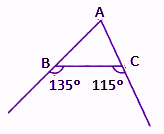Solution:

135° and ∠ABC form a linear pair.

∠ABC = 180° – 135° = 45°

Similarly,

∠ACB = 180° – 115° = 65°

Here, ∠ACB > ∠ABC

Therefore, AB > AC

Question 13: In any triangle ABC, ∠A > ∠B and ∠B > ∠C, then what will be the smallest side?

Solution:

In triangle ABC,

∠A > ∠B and ∠B > ∠C

That means,

∠A > ∠C

or

∠C < ∠B < ∠A

Since ∠C is the least angle, side AB will be the smallest side.

Question 14: P is any point lie on the bisector of ∠ABC. If through P, a line is drawn parallel to BA meets line BC at Q, then prove that ΔBPQ is an isosceles triangle.

Solution: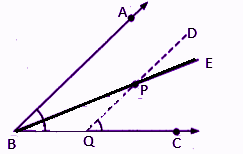P lies on the bisector of ∠ABC.

DQ is parallel to AB.

∠ABC = ∠DQC (corresponding angles)

∠ABP + ∠PBC = ∠DQC

And BE is the bisector of ∠ABC.

∠ABP = ∠PBC

∠DQC = ∠PBC + ∠BPQ (by exterior angle property)

∠ABP + ∠PBC = ∠PBC + ∠BPQ

∠ABP = ∠BPQ

∠PBC = ∠BPQ (since ∠ABP = ∠PBC)

That means, QP = BQ

Therefore, ΔBPQ is an isosceles triangle.

Question 15: ABC is a right angled triangle in which AB = AC. Bisector of ∠A meets BC at D. Prove that BC = 2AD.

Solution: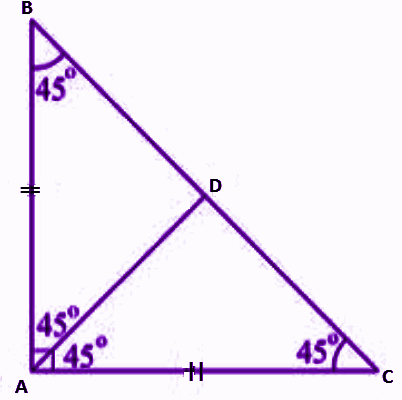In ΔABC right angled at A and AB = AC

Therefore, ∠ A = ∠ B

We know that Sum of all the three angles of a triangle = 180°

∠A + ∠B + ∠C = 180°

90° + ∠B + ∠B = 180º

2∠B = 180° – 90°

2∠B = 90°

∠B = 45° ….(i)

And AD is the bisector of ∠BAC.

SO, AD = BD .…(iii) .

Hence proved.

Question 16: ΔABC and ΔDBC lie on the same base BC such that points A and D are on the opposite side of BC and AB = AC and DB = DC. Show that AD is the perpendicular bisector of BC.

Solution: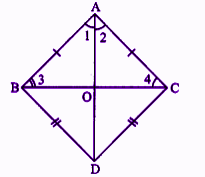AB = AC (given)

BD = DC (given)

∠1 = ∠2 (by CPCT)

Also, AB = AC (given)

We know that angles opposite to equal sides are equal.

∠3 = ∠4

∆BAO ≅ ∆CAO

⇒ BO = OC (by CPCT)

or

AO bisects BC (by CPCT)

Also, ∠AOB = ∠AOC (by CPCT)

We know that ∠AOB + ∠AOC = 180°

⇒ ∠AOB = ∠AOC = 90°

Therefore, AD is a perpendicular bisector of BC.

Question 17: Prove that the sum of any two sides of a triangle is greater than twice the median drawn to the third side.

Solution:

Given: In ∆ABC,

To prove: AB + AC > 2AD

Join EC.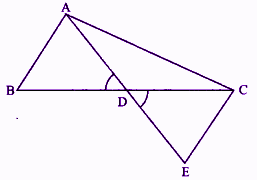AD = DE (by the construction)

BD = DC (given)

and ∠ADB = ∠EDC (vertically opposite angles)

∆ADB = ∆EDC (by SAS congruence criterion)

⇒ AB = CE (by CPCT)

In ∆ACE,

AC + CE > AE

⇒ AC + AB > AE (∵ AB = CE) (Proved above)

Hence proved.

Question 18: In ∆ABC, D is the midpoint of side AC such that BD = 1/2 AC. Show that ∠ABC is a right angle.

Solution:

Given that D is mid-point of AC.

⇒ AD = DC = 1/2 AC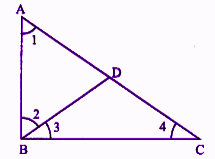And BD = 1/2 AC (given)

⇒ AD = DC = BD

In ∆ABD,

Let ∠2 = ∠1 = x (angles opposite to equal sides are equal)

Also, BD = DC

∠3 = ∠4 = x

In ∆ABC,

∠1 + (∠2 + ∠3) + ∠4 = 180°

x + (x + x) + x = 180°

4x = 1 80°

x = 45°

∠ABC = x + x = 45° + 45° = 90°

That means ∠ABC is a right angle.

Hence proved.

Question 19: Prove that the line segment joining the midpoint of the hypotenuse of a right triangle to the vertex of the right angle is equal to half the hypotenuse.

Solution:

Let ABC be a right triangle right angled at B.

Let P be the mid-point of the hypotenuse AC.

Through P, draw PQ || BC.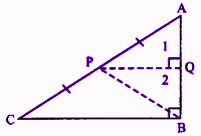PQ || BC

⇒ ∠1 = ∠B (corresponding angles)

⇒ ∠2 = ∠1 = 90°

In ∆ABC, P is the mid-point of AC and PQ || BC.

Hence, Q must be midpoint of AB (by the converse of mid-point theorem)

⇒ AQ = QB ….(i)

Now in ∆AQP and ∆BPQ,

AQ = QB

∠2 = ∠1 = 90°

PQ = PQ

∆AQP ≅ ∆BPQ (by SAS congruence criterion)

⇒ AP = PB (by CPCT)

Therefore, AP = PB = PC

We can say that the point P is equidistant from the vertices of a right angled triangle ABC.

Hence proved.

Question 20: In the figure, if AB = AC, then write the relation between AB and AD.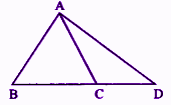Solution:

Given that, AB = AC

Therefore, ABC is an isosceles triangle.

BC is produced to D.

Hence, AD is greater than AB.

Question 21: AD is the median of ∆ABC. Is it true that AB + BC + CA > 2AD. Give reason for your answer.

Solution: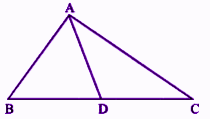Yes, if AD is median of ∆ABC:

AB + BD > AD ….(i) (sum of any two sides of a A is greater than third side)

Similarly,

AC + DC > AD ….(ii)

AB + (BD + DC) + AC > 2 AD

AB + BC + CA > 2AD

Question 22: M is any point on the side BC of ∆ABC such that AM is the bisector of ∠BAC. Is it true that the perimeter of ∆ABC is more than 2 AM? Give reason for your answer.

Solution: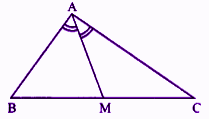Yes, AB + BM > AM ….(i)

Similarly,

AC + CM > AM ….(ii)

AB + (BM + CM) + AC > 2AM

AB + BC + CA > 2AM

Therefore, Perimeter of ∆ABC > 2AM

Question 23: Q is a point situated on the side SR of ∆PSR such that PQ = PR. Prove that: PS > PQ

Solution: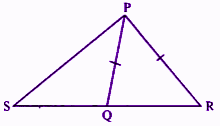In ∆PQR,

PQ = PR

∠PQR = ∠PRQ ….(i) (angles opposite to equal sides of a triangle are equal)

In ∆PSQ,

Exterior of ∠PQR > ∠PSQ ….(ii)

From (i) and (ii),

∠PRQ > ∠PSQ

⇒ PS > PR (side opposite to greater angle is longer)

⇒ PS > PQ (∵ PQ = PR)

Hence proved.

Question 24: S is any point situated on the side QR of ΔPQR. Show that PQ + QR + RP > 2PS.

Solution:

In ∆PQR,

PQ + QS > PS ….(i) (the sum of any two sides of a triangle is greater than the third side)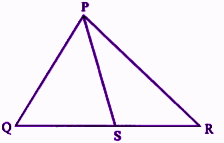Similarly,

In ∆PRS,

PR + RS > PS …(ii)

(PQ + QS) + (PR + RS) > 2PS

PQ + (QS + RS) + PR > 2PS

PQ + QR + PR > 2PS

PQ + QR + RP > 2PS

Hence proved.

Question 25: D is any point situated on side AC of ΔABC, with AB = AC. Show that CD < BD.

Solution:

In triangle ABC,

AB = AC (given)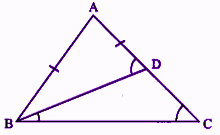⇒∠B = ∠C

⇒ ∠ADB = ∠DBC + ∠C (exterior angle property)

⇒ AB > BD

But AB = AC (given)

⇒ AD + DC > BD

⇒ BD > CD

Hence proved.

Question 26: In the figure, ∠B > ∠A and ∠D > ∠E then prove that AE > BD.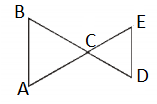Solution:

Given,

∠B > ∠A

⇒ AC > BC ….(i)

Also ∠D > ∠E

CE > CD ….(ii) (greater angle has larger side opposite to it)

AC + CE > BC + CD

⇒ AE > BD

Hence proved.

Question 27: In ΔABC, AB > AC and D is any point on the side BC. Prove that AB > AD.

Solution:

In ∆ABC,

AB > AC (given)

⇒ ∠ACB > ∠ABC …(i) (angle opposite to larger side is greater)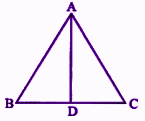Now in ∆ACD, CD is produced to B forming an exterior of ∠ADB.

∠ADB > ∠ACD (exterior angle of a A is greater than each interior opposite angle)

∠ACD = ∠ACB

From (i) and (ii),

⇒ ∠ADB > ∠ABD (∠ABC = ∠ABD)

⇒ AB > AD (side opposite to greater angle is larger)

Hence proved.

Question 28: Prove that the sum of three sides of a triangle is greater than the sum of its three medians.

Solution:

Let us assume that, in the ∆ABC, AD, BE and CF be its medians.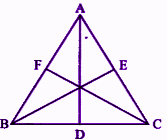We know that the sum of any two sides of a triangle is greater than twice the median bisecting the third side.

Therefore, AD is the median bisecting BC.

⇒ AB + AC > 2AD ….(i)

BE is the median bisecting AC.

⇒ AB + BC > 2BE ….(ii)

Similarly, for median CF.

BC + AC > 2CF ….(ii)

2 (AB + BC + CA) > 2 (AD + BE + CF)

⇒ AB + BC + CA > AD + BE + CF

Hence proved.

Question 29: In the figure, O is the interior point in a triangle ABC, then prove that: BC + AB + CA < 2(OA + OB + OC).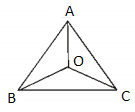Solution:

Given that, in triangle ABC, O is the interior point.

We know that the sum of any two sides of a triangle is greater than the third side.

In triangle AOB,

OA + OB > AB ….(i)

In triangle AOC,

OA + OC > AC ….(ii)

In triangle BOC,

OB + OC > BC ….(iii)